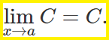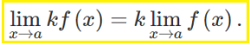# Properties of Limits (Limit Laws)

The title might sound daunting, but properties of limits (also called limit laws) are just shortcuts to finding limits of functions.

## How To Use Properties of Limits

To find a limit using the properties of limits rule:

1. Figure out what kind of function you are dealing with in the list of “Function Types” below (for example, an exponential function or a logarithmic function),
2. Click on the function name to skip to the correct rule,
3. Substitute your specific function into the rule.

## Function Types

Click a function name in the left column to skip to that rule.

 Rule Name Notation Example 1. Constant function f(x) = C y = 5 2. Constant multiplied by another function k * f(x) 5 * 10x2 3. Sum of functions f(x) + g(x) + … 10x2 + 5x 4. Product of two or more functions f(x) * g(x) * … 10x2 * 5x 5.Quotient Law f(x) / g(x) 5x / 10x2 6. Power functions f(x) = axp 10x2 7. Exponential functions f(x) = bx 10x 8. Logarithmic functions f(x) = logbx log10x

## 1. Constant FunctionThe limit of a constant function C is equal to the constant.

Example: if the function is y = 5, then the limit is 5.

## 2. Constant Multiplied by a Function (Constant Multiple Rule)The limit of a constant (k) multiplied by a function equals the constant multiplied by the limit of the function.Limit of 5 * 10x2 as x approaches 2.Example: Find the limit of f(x) = 5 * 10x2 as x→2.

1. The limit of f(x) = 5 is 5 (from rule 1 above).
2. The limit of 10x2 at x = 2 can be found with direct substitution (where you just plug in the x-value): 10((22) = 40
3. Multiply your answers from (1) and (2) together: 5 * 40 = 200

Tip: Plot a graph (using a graphing calculator) to check your answers.

## 3. Sum of FunctionsThe limit of a sum equals the sum of the limits. In other words, figure out the limit for each piece, then add them together.

For step by step examples, see: Sum rule for limits.

## 4. Product of Two or More FunctionsThe limit of a product (multiplication) is equal to the product of the limits. In other words, find the limits of the individual parts and then multiply those together.

Example: Find the limit as x→2 for x2 · 5 · 10x

1. The limit of x2 as x→2 (using direct substitution) is x2 = 22 = 4
2. The limit of the constant 5 (rule 1 above) is 5
3. Limit of 10x (using direct substitution again) = 10(2) = 20
4. Multiply (1), (2) and (3) together: 4 · 5 · 20 = 400

## Extended Product RuleAny “extended” formulas in properties of limits are just extensions of other formulas. This one is just an extension of the product rule above: you can just keep on multiplying as many parts as you need (e.g. a * b * c * d * …).

## 5. Quotient of Two or More FunctionsThe limit of a quotient is equal to the quotient of the limits. In other words:

• Find the limit for the numerator,
• Find the limit for the denominator,
• Divide the two (assuming that the denominator isn’t zero!).

## 6. Power FunctionsThe rule for power functions states: The limit of the power of a function is the power of the limit of the function, where p is any real number.Example: Find the limit of the function f(x) = x2 as x→2.

1. Remove the power: f(x) = x
2. Find the limit of step 1 at the given x-value (x→2): the limit of f(x) = 2 at x = 2 is 2. You can use direct substitution or a graph like the one on the left.
3. Put the power back in: 22 = 4

A particular case involving a radical:Also, if f(x) = xn, then:This particular part of the properties of limits “rule” for power functions is really just a shortcut: The limit of x power is a power when x approaches a.

## 7. Exponential Functions## 8. Logarithmic Functions## Properties of Limits: References

Gunnels, P. (undated). Limit Laws. Retrieved May 29, 2019 from: http://people.math.umass.edu/~gunnells/teaching/Sample_Lecture_Notes.pdf

CITE THIS AS:
Stephanie Glen. "Properties of Limits (Limit Laws)" From StatisticsHowTo.com: Elementary Statistics for the rest of us! https://www.statisticshowto.com/limit-of-functions/properties-of-limits-laws/